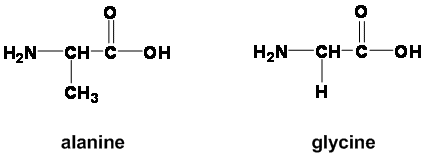# Why is the pKa (COOH) of alanine and glycine similar but the pKa (NH3) different?

Alanine is heavier with a ($\ce{-CH3}$) than glycine which has an ($\ce{-H}$). What makes them have different $\mathrm{p}K_\text{a}(\ce{-NH3+})$ $(\mathrm{p}K_\text{a}: \text{Ala}=9.69, \text{Gly}=9.60)$ but similar $\mathrm{p}K_\text{a}(\ce{-COOH})$ $(\mathrm{p}K_\text{a}=2.34)$ when the rest of the amino acid is similar? Why does changing a hydrogen for a methyl group affect the $\ce{-NH3+}$ but not the $\ce{-COOH}$?Also there are three amino acids (glycine, leucine, aspartine) which have same $\mathrm{p}K_\text{a}(\ce{-NH3+})$ but different $\mathrm{p}K_\text{a}(\ce{-COOH})$.

• Can you add the pKas for both amino acids? Jan 11, 2015 at 21:32

I found the following pKa values for glycine and alanine from the CRC Handbook of Chemistry and Physics via Sigma-Aldrich:

Glycine $\ce{COOH}$: 2.34
Alanine $\ce{COOH}$: 2.34

Glycine $\ce{NH3+}$: 9.60
Alanine $\ce{NH3+}$: 9.69

First, although the values for the carboxylic acid are identical as reported, you would see a difference if you found a value reported with enough precision. The carboxylic acid is not immune to changes in the structure of the rest of the molecule.

Second, the difference between 9.60 and 9.69 is pretty small. In most practical situations, the two amino acids would behave identically with regard to the $\ce{NH3+}$ pKa.

The fact that the magnitude of the difference is larger for the $\ce{NH3+}$ pKa values can likely be attributed to proximity. In this case, the relevant proton is 2 bonds away from the $\alpha$ carbon, whereas the proton in the $\ce{COOH}$ group is 3 bonds away.